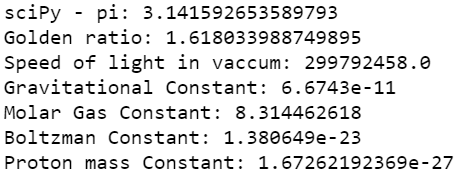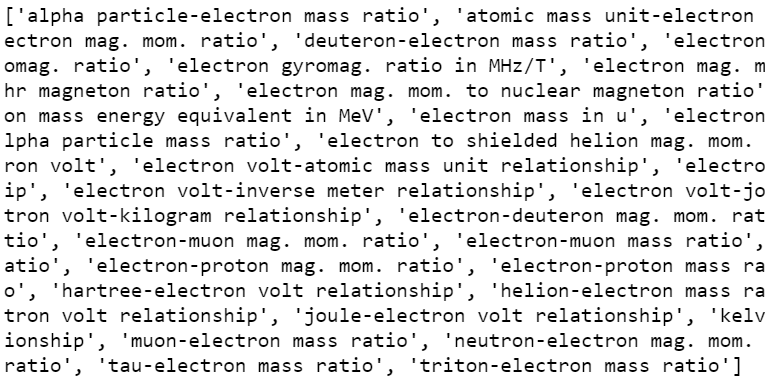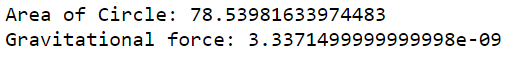GeeksforGeeks App
Open AppBrowser
Continue

# SciPy – Constants

Scipy stands for Scientific Python and in any Scientific/Mathematical calculation, we often need universal constants to carry out tasks, one famous example is calculating the Area of a circle = ‘pi*r*r’ where PI = 3.14… or a more complicated one like finding forcegravity = G*M*m ⁄ (distance)2 where G = gravitational constant. In all such scenarios, it would be very handy if we have reference material to look up these constants and incorporate them into our calculation with ease.

Scipy-Constants is a sub-module inside the Scipy library that does this for us. It contains an exhaustive list of universal mathematical constants, Physical constants, and units. which can be looked up with just 1 line of code.

### Accessing Constants

Just type the name of the constant in place of XXXX in ‘scipy.constants.XXXX‘ format to access its value. Below listed are a few most important constants using scipy.constant module down below. The list is not exhaustive, but it gives a good idea of how to access constants.

## Python3

 `# import module``import` `scipy` `# Just type the name of the constant in``# scipy.constant.XXXX format to access its value.``print``(``"sciPy - pi:"``, scipy.constants.pi)``print``(``"Golden ratio:"``, scipy.constants.golden_ratio)``print``(``"Speed of light in vacuum:"``, scipy.constants.c)``print``(``"Gravitational Constant:"``, scipy.constants.G)``print``(``"Molar Gas Constant:"``, scipy.constants.R)``print``(``"Boltzman Constant:"``, scipy.constants.k)``print``(``"Proton mass Constant:"``, scipy.constants.proton_mass)`

Output:### Finding Constants

We can use an inbuilt method to find the constants relevant to our use case. Constants are stored using a dictionary data structure, and we can use the scipy. constants.find() API to find all relevant constants from the dict and use them accordingly.

The code below demonstrates using scipy. constants.find() API. The below code prints all constants which contain the ‘electron’ word in it, and we can filter out the one which is required.

## Python3

 `import` `scipy`  `# find method looks up in the dictorary and``# finds out all the constants containing``# 'electron' word in it and returns a list``# of constants.``res ``=` `scipy.constants.find(``"electron"``)``print``(res, end``=``'\n'``)`

Output:Not just the magnitude of a constant we can also access the unit and degree of uncertainty associated with the magnitude of any physical_constants stored in scipy.constants module, using the format

physical_constants[name] = (value, unit, uncertainty).

## Python3

 `import` `scipy`  `# This returns a tuple (value, unit, uncertainty)``# associated with the physical constant``print``(scipy.constants.physical_constants[``'alpha particle mass'``])`

Output:

`(6.6446573357e-27, 'kg', 2e-36)`

Example:

## Python3

 `import` `scipy` `# Area of a circle using``# scipy.constants.pi``def` `Area_of_Circle(r):``    ``return` `scipy.constants.pi ``*` `r ``*` `r` `# Calculates the gravational for``def` `force_gravity(M, m, dist):``    ``return` `(scipy.constants.G``*``M``*``m) ``/` `(dist``*``*``2``)`  `print``(f``'Area of Circle: {Area_of_Circle(5)}'``)``print``(f``'Gravitational force: {force_gravity(10,5,1)}'``)`

Output:Apart from the above variables, scipy.constants also contain more physical constants, and below is a list of all methods available in scipy.constants module with an explanation.

Below are the most commonly used constants available in SciPy module:

Below are the unit constants available in the SciPy module:

• Mass:
• Time:
• Length:
• Pressure:
• Area:
• Speed:

My Personal Notes arrow_drop_up